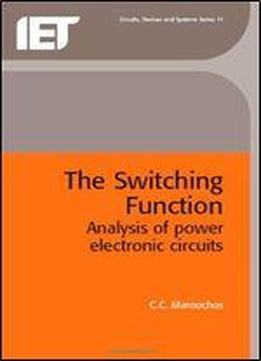# Theswitching Function: Analysis Of Power Electronic Circuits by C.C. Marouchos / 2006 / English / PDF

This book demonstrates the usefulness of the switching function in analyzing powers electronic circuits in the steady state. A procedure is suggested for the effective application of this effective application of this method for the analysis of all types of power electronic circuits. The Kirchoff's Laws and the Superposition theorem are applied by introducing the appropriate switching functions in order to derive Unified Expressions of voltage and current in switched circuits valid at all times. The exact expressions of the current in each semiconductor device in the circuit enables the circuit designer to collect all the relevant data to set the ratings of the device such as rms, average and peak values of voltage and current. The order of the voltage and current harmonics at any point in the circuit are derived with simple arithmetic. Compact expressions are derived for Sinusoidal PWM signals based on the switching function. The order, magnitude and phase of each component are derived directly from the expression with simple arithmetic. The educator has a simple way to present to his students the mechanism of operation of complex switched circuits where all the statements regarding their operation are actually presented in the model of the circuit.

views: 128